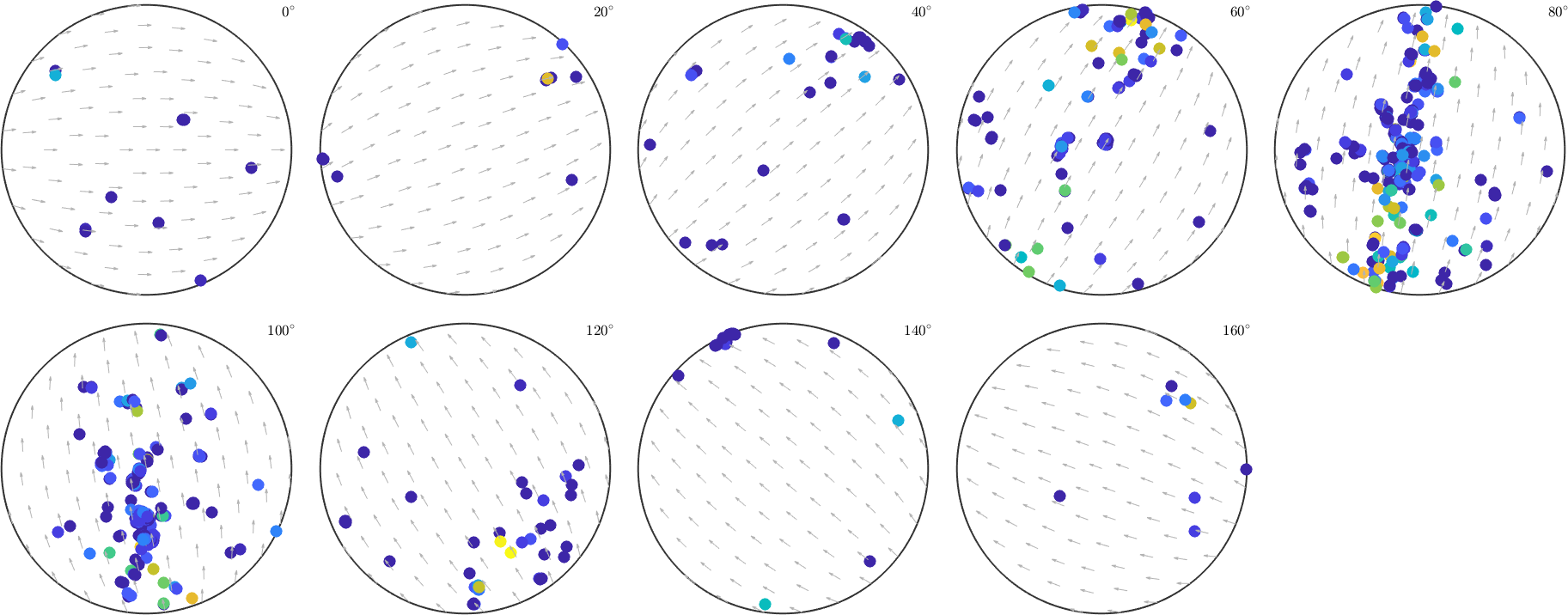Plotting Individual Orientations edit page

Basics of the plot types for individual orientations data

This section gives an overview over the possibilities that MTEX offers to visualize orientation data. Let us first load a sample EBSD data set

and select all individual orientations of the Iron phase

## Scatter Pole Figure Plot

A pole figure showing scattered points of these data figure can be produced by the command plotPDF.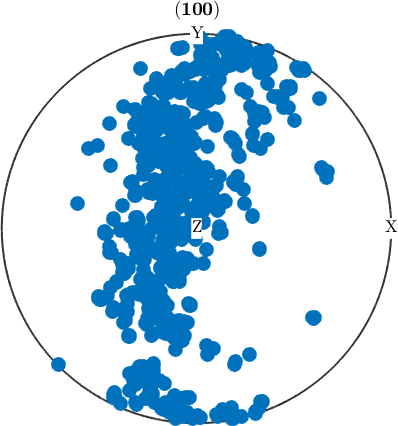## Scatter (Inverse) Pole Figure Plot

Accordingly, scatter points in inverse pole figures are produced by the command plotIPDF.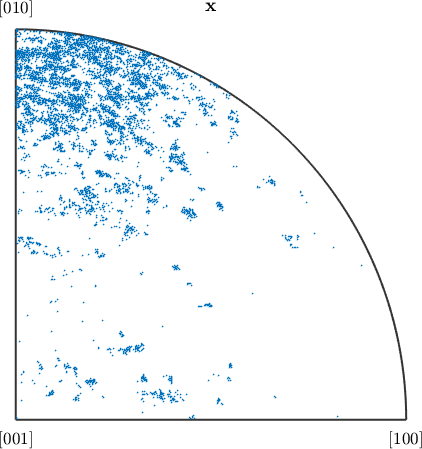## Scatter Plot in ODF Sections

The plotting of scatter points in sections of the orientation space is carried out by the command plotSection. In the above examples, the number of plotted orientations was chosen automatically such that the plots not to become too crowded with points. The number of randomly chosen orientations can be specified by the option points.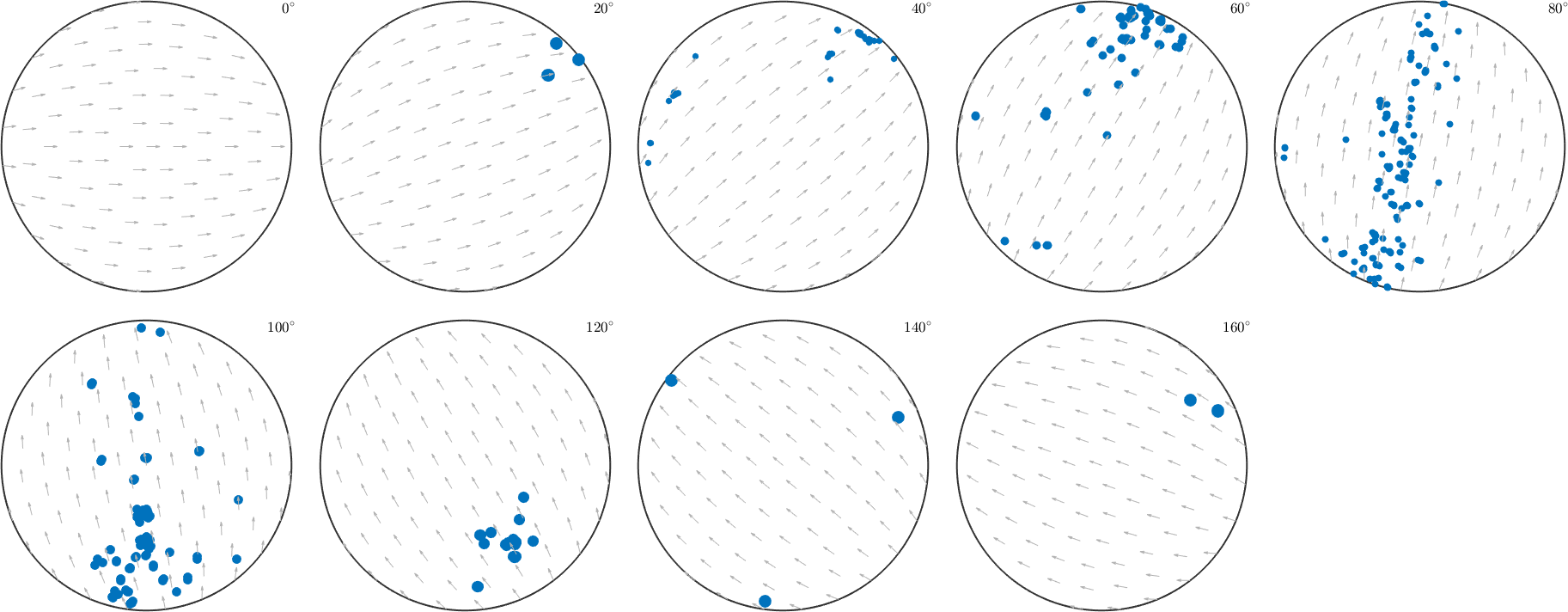## Scatter Plot in Axis Angle or Rodrigues Space

Another possibility is to plot the single orientations directly into the orientation space, i.e., either in axis/angle parameterization or in Rodrigues parameterization.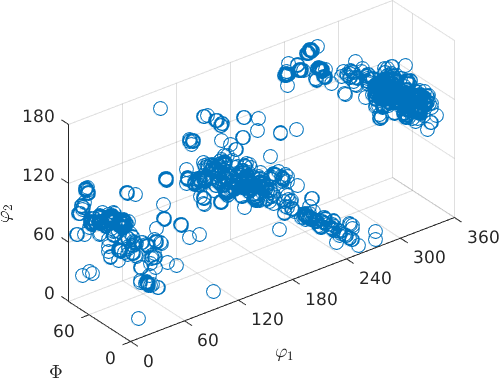Here, the optional option 'center' specifies the center of the unique region in the orientation space.

## Orientation plots for EBSD and grains

Since EBSD and grain data involves single orientations, the above plotting commands are also applicable for those objects.

Let us consider some grains reconstructed from the EBSD data

Then the scatter plot of the individual orientations of the Iron phase in the inverse pole figure is achieved by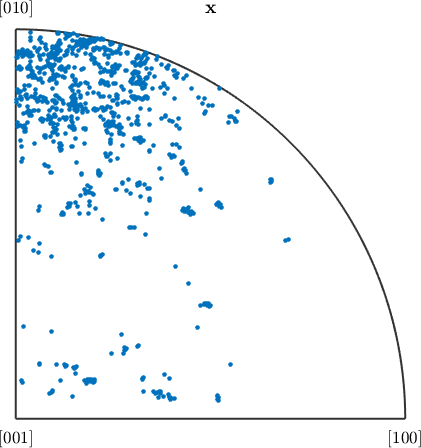In the same way, the mean orientations of grains can be visualized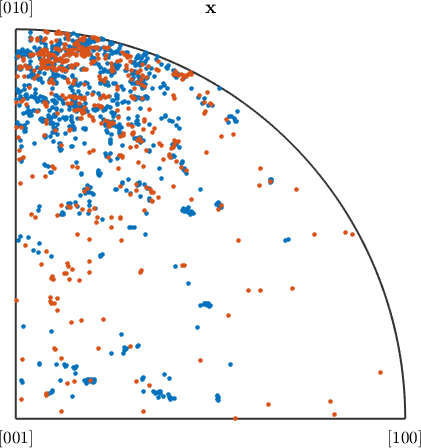One can also use different colors on the scatter points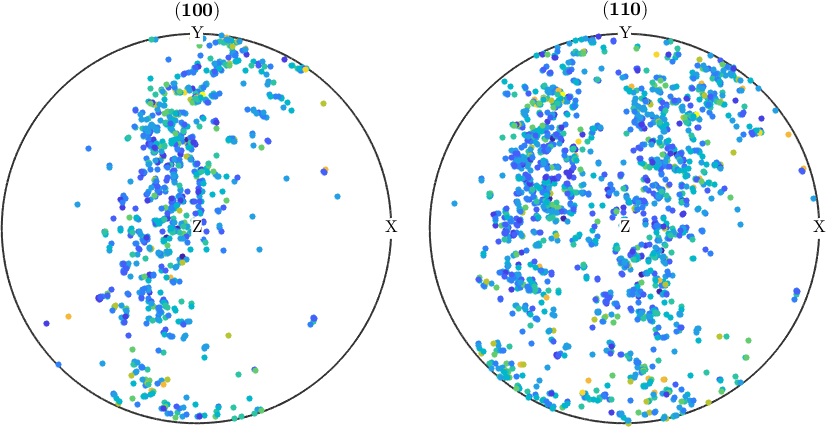or some arbitrary data vector